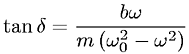Equations > Physics > Oscillations and Waves > Phase constant of a driven oscillation

### Phase constant of a driven oscillationLatex Code:

MathML Code:

 $\mathrm{tan}\delta =\fracb\omega m\left({\omega }_{0}^{2}-{\omega }^{2}\right)$

MathType 5.0: